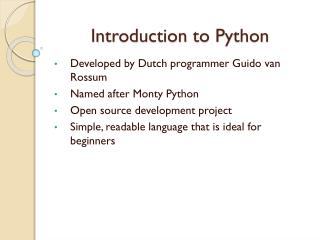DownloadDownload PresentationIntroduction to Python

# Introduction to Python

Download Presentation## Introduction to Python

- - - - - - - - - - - - - - - - - - - - - - - - - - - E N D - - - - - - - - - - - - - - - - - - - - - - - - - - -
##### Presentation Transcript

1. Introduction to Python Developed by Dutch programmer Guido van Rossum Named after Monty Python Open source development project Simple, readable language that is ideal for beginners

2. Python Software • Software may be downloaded from: http://www.python.org/download/ • Download the Python 3.x version • An IDE (IDLE IDE) is included in the standard implementation

3. Getting Started….. • Basic Arithmetic Operators • +, -, /, *, % • // is used for integer division (Example: 9 // 4 will give you 2) • ** is used for exponentiation ( 2**3 gives 8) • Some simple functions: • abs(), min(), max()

4. Boolean Expressions/Operators • Boolean values: True, False • and (&& in Java), or (|| in Java), not (! In Java) • Comparisons: >, >=, <, <=. ==, !=

5. Variable Names • A through Z, lowercase or uppercase, underscore, and digits 0-9 (except for the first character) • Variable name cannot start with a digit • Examples: account, account63, bank_account, bankAccount, … • Starting with Python 3, characters in other languages may be used (Unicode characters) • Variables in Python don’t have a type

6. Strings • Enclosed in quotes (single or double may be used) • s=‘Hello!’ is an example of a string assignment • s==‘Hello!’ evaluates to True • s==‘hello!’ evaluates to False • s=s + ‘ How are you?’ (String concatenation)

7. More on strings…. • x=‘bonjour’ • len(x) gives 7, the length of the string • ‘on’ in x evaluates to True (‘on’ is a substring of x) • x gives ‘b’ (the character at index 0) • x * 2 will give ‘bonjourbonjour’ • x[-1] displays ‘r’, the last character • x[-2] displays ‘u’, the last but one character

8. Lists • Stores a sequence of objects • books = [‘The Jungle Book’, ‘The Idiot’, ‘The Razor’s Edge’] • [ ] is an empty list • books displays ‘The Jungle Book’ • Books[-1] displays ‘The Razor’s Edge’ • Lists can contain lists • Example: x = [ 1, [2, 3], 4] • x displays [2, 3]

9. Some operators and functions • + to concatenate lists • [1,2] * n will repeat 1,2 n times • If x = [1,2,3,4], then: • 3 in x will evaluate to True • 5 not in x will be True • sum(x) will be 10 (sum of items in list) • max(x) will be 4, min(x) will be 1

10. More on lists • Lists are mutable, strings are not • colors = [‘red’, ‘blue’, ‘green’] • colors.append(‘yellow’) appends ‘yellow’ to colors • colors.count(‘red’) returns 1, the number of occurrences of ‘red’ in the list • colors.remove(‘blue’) removes first occurrence of ‘blue’ • colors.reverse() reverses the list • color.sort() sorts in ascending order

11. Math Module • Module is similar to the notion of a package • import math to use math functions • Math functions/constants include sqrt(), ceil(), floor(), cos(), sin(), log(), pi, e

12. Turtle Graphics #Turtle graphics #This program uses two Turtle objects to draw random lines on the #screen import turtle import random #instantiate the Screen object on which we will be drawing s=turtle.Screen() #first Turtle object turtle1 = turtle.Turtle() turtle1.pencolor('red') turtle1.pendown() turtle2.forward(100)

13. Useful Built-In Functions • print() • input() • Example: name = input(“What is your name? “) print(“Hello “ + name + “, how are you?”)

14. If statement • Syntax: if <condition>: <code should be indented> elif<condition>: <code under this condition> else: <code for the last else>

15. If Example age = int(input(“Enter your age: “)) if age < 10: print(“You are rather young for Python”) else: print(“Conquer the world with Python”)

16. Iteration • For animals = [‘dogs’, ‘cats’, ‘lions’] #iterate through list and display animals for x in animals: print(x) • While See example on next slide…

17. Example of while validNames = [‘Don’, ‘Peter’, ‘Mary’] loginName = input(“Enter login name: “) #stay in loop until user enters the right #user name while loginNamenot in validNames: loginName = input(“Invalid name! Try again: “) print(“You are in!”)

18. User-Defined Functions defdisplayMessage(): print(“Hi there! Have a nice day”) def sum(x, y): return x + y displayMessage() print(sum(3,8))

19. Strings, substrings s = “goodbye!” What is s? (Ans: ‘g’) s[0:4] would be ‘good’ s[:4] is also ‘good’ s[3:7] would be ‘dbye’ s[4:] would give you ‘bye!’ s[-1] is ? (Ans: ‘!’) s[-3: ] would be ‘ye!’

20. Some string methods • s = ‘bonjour’ • s.find(‘on’) returns 1 (first occurrence of ‘on’) • s.upper()  ‘BONJOUR’ • s.lower()  ? • s.replace(‘o’, ‘i’)  ‘binjiur’ • s.strip() gets rid of leading/trailing blanks